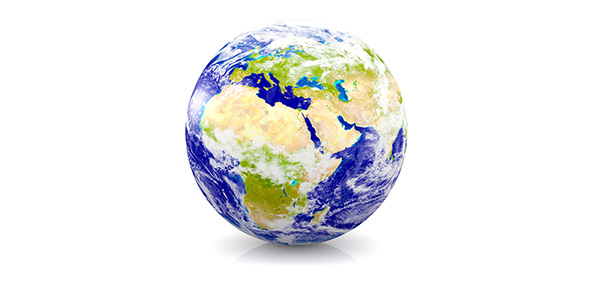# How Much Can You Score On This Earth Quiz?

27 Questions | Total Attempts: 216SettingsIt's time to answer some interesting questions on Earth! Let’s take this quiz and find out how much you really know.

• 1.
What is the name for the huge landmass that Alfred Wegener believed covered much of the Earth 200 million years ago?
• A.

Pangea

• B.

Mantle

• C.

Crust

• D.

The Alotaland

• 2.
What kind of boundary is formed when two lithospheric plates slide past each other?
• A.

Oceanic Boundary

• B.

Crust Boundary

• C.

Transform Boundary

• D.

Green Boundary

• 3.
Which of the following are layers of the Earth?
• A.

Precipitation, moderation, epitaph, graphic

• B.

Inner, Outer, Mantle, Crust

• C.

Jesuit, Epinephrin, Asprin, Decongestant

• D.

• 4.
What two types of crust make up the surface of the earth?
• A.

Continental & Osmosis

• B.

Continental & Divide

• C.

Oceanic & Oleander

• D.

Oceanic & Continental

• 5.
How did the Himalayan Mountains form?
• A.

Two continental plates converged

• B.

Two continental plates diverged

• C.

Two oceanic plates converged

• D.

An oceanic plate and a continental plate converged

• 6.
As rock moves away from a mid - ocean ridge, it is replaced by:
• A.

Molten rock

• B.

Continental crust

• C.

Suspect terraines

• D.

Older rock

• 7.
The region along a plate boundary where one plate is forced under another plate is called a:
• A.

Rift valley

• B.

Paleomagnetic band

• C.

Transform fault

• D.

Subduction zone

• 8.
• A.

Subduction zones

• B.

Divergent boundaries

• C.

Transform faults

• D.

Terraine boundaries

• 9.
If you used the theory of plate tectonics to predict the most likely place for the next earthquake or volcanic eruption , you should predict that it is most likely to occur:
• A.

Where one has not happened in at least 10 million years

• B.

In the interior of any continent

• C.

Along boundaries between colliding lithospheric plates

• D.

Where two plates are diverging from one another.

• 10.
__________________ means "shrinking" or decreasing in illumination
• A.

Waining

• B.

Waxing

• C.

Weathered

• D.

Warrior

• 11.
__________________ means "growing" or expanding in illumination
• A.

Weathered

• B.

Warrior

• C.

Waning

• D.

Waxing

• 12.
When the Moon is between the Earth and the Sun, it is most likely what phase?
• A.

Full Moon

• B.

1st Quarter

• C.

New Moon

• D.

3rd Quarter

• 13.
During the New Moon phase, we can see light from the Sun shining on the Moon
• A.

True

• B.

False

• C.

Maybe

• D.

IDK

• 14.
Choose the correct order of the Moon Phases.
• A.

Choose the correct order of the Moon Phases.

• B.

1st Quarter, 3rd Quarter, New, Full

• C.

New, 1st Quarter, Full, 3rd Quarter

• D.

New, Full, 1st Quarter, 3rd Quarter

• 15.
Why does the Moon have different phases?
• A.

It moves around the Sun

• B.

The Sun moves around it

• C.

It moves around the Earth

• D.

The Earth moves around it

• 16.
About how long does it take the Moon to complete it's cycle?
• A.

1 Year

• B.

1 Month

• C.

1 Century

• D.

1 Week

• 17.
The moon gets its light from:
• A.

Mercury

• B.

Venus

• C.

The Sun

• D.

The Stars

• 18.
When the Earth is between the Moon and the Sun, it is most likely a:
• A.

Full Moon

• B.

1st Quarter Moon

• C.

New Moon

• D.

3rd Quarter Moon

• 19.
What size is the moon compared to the Earth
• A.

Bigger

• B.

Smaller

• C.

Same Size

• D.

The Moon Does Not Exist

• 20.
_________________ is defined when an object’s distance from another object ischanging.
• A.

Speed

• B.

Motion

• C.

Force

• D.

Size

• 21.
What is the formula for speed?
• A.

Speed = Force + Motion

• B.

Distance X Foce = Speed

• C.

Time + Distance = Speed

• D.

Speed=Distance / Time

• 22.
In physical science, Force is:
• A.

A push or pull

• B.

A left or right

• C.

A measure of time

• D.

A measure of height

• 23.
____________________ is defined as total distance divided by the total time?
• A.

Average Weight

• B.

Average Size

• C.

Average Speed

• D.

Average Distance

• 24.
A Newton is used to measure ______________
• A.

Motion

• B.

Force

• C.

Distance

• D.

Speed

• 25.
A(n) ________________________ force allows an object to move.
• A.

Unbalanced

• B.

Balanced

• C.

Equal

• D.

Puppy

Related TopicsBack to top